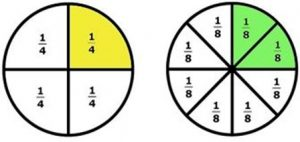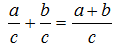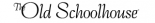Fractions say, “I have an equivalent.”

Fractions say, “Look at my denominator before you add.”

Fractions say, “Look at my denominator before you subtract.”

Fractions say, “Multiply numerator times numerator and denominator times denominator, in order to multiply.”

Fractions say, “Invert the second term then follow rules for multiplication, if you must divide.”

When you see your child struggling with algebra, you do all you can to ease him into a friendly comfort zone. Often, it is difficult for a child to pinpoint exactly what causes him to “just not get it.” Once he emerges from the confusion of alphabets parading as numbers, he feels safely on his way to understanding algebra. Unfortunately, he keeps bumping into “4/5 c” or “2/3 d”. These fractions occur everywhere he turns; number properties have them, radicals have them, polynomial expressions have them, functions have them, equations have them, inequalities have them as well. How can he make it through the course? At this point, it strikes you that fractions are holding back your child from moving further into algebra. He cannot understand algebra because he does not understand fractions. You say to him, “Understand fractions to understand algebra.”

To clear the ground of fraction difficulties, you look around for specific areas where trouble might brew. What jumps out at you immediately is the area of equivalent fractions.

Equivalent fractions are fractions that rename another fraction. They are fractions that have the same value. If one fraction is reduced, you get its equivalent fraction. If you find the least common denominator for two or more fractions during addition or subtraction, you carry out the operation by changing the fractions into equivalent fractions.Recognition 1: Refresh study of equivalent fractions, if one currently takes algebra and quarrels with fractions.

Look again. After equivalent fractions, take a peek to examine addition or subtraction of fractions and start with polynomial expressions. Coming up early in algebra studies, polynomial expressions require learners to group like terms together, and then simplify. Grouping like terms means that you gather all the a’s together, all the b’s together, and all the c’s together; put all apples in basket one, put all bananas in basket two, and put all cupcakes in basket three.

Now, you must add them or subtract them:

(5/9 a + 2/9 a) – (3/8 b + 2/8 b) = 7/9 a – 5/8 b

Although grouping and simplifying like terms highlights the algebra, a learner may be thrown off path by the need to add or subtract fractions. Here, one must learn or relearn how to add or subtract fractions not only for the sake of simplifying polynomial expressions, but for the sake of equations, inequalities, functions, radicals, quadratic equations, and other algebra concepts.

Most frequently, it is the familiarity with the rule for denominators that determines how well addition and subtraction are handled. Simply stated, denominators must be the same for all fractions involved in addition or subtraction. If denominators are not the same, then learners must find the LCD (Least Common Denominator) and change the fractions before proceeding with the operation.

Recognition 2: Rules for adding or subtracting fractions remain important in algebraic expressions of any kind, in equations, and in inequalities.Look again: Multiplication or division of fractions takes strong footing on algebra’s mountains of equations, inequalities, and other concept valleys.

If you consider a simple equation which you were solving for one variable, your strategy probably led you to do the same to both sides of the equation in order to isolate the variable to one side of the equation. This means that you added, subtracted, multiplied, or divided the same number on both sides of the equation. The number might be a fraction. If the learner already knows how to use these fraction operations, his attention will bend toward finding the variable. He will not veer off the path to learn or relearn the how-to of operating with fractions.

5X + 3/5 = 30 3/5

5X  +  3/5  —  3/5  =  30 3/5  —  3/5  >>>> Here, you subtracted 3/5 from both sides of the equation.

5X = 30 >>>>>>>>> Result of subtracting—it’s a simple multiplication problem.

5X/5 = 30/5 >>>>>> Here, both sides of the equation divided by 5.

X = 6 >>>>>>>>>> Here, the variable isolated to one side of the equation. Equation solved.

Recognition 3: Multiplication and division of fractions are critical to problem solving in algebra.

In essence, whether determining how to make equivalent fractions in any type of algebra problem, simplifying polynomial expressions through addition or subtraction, or solving for one variable in an equation that may use multiplication and division, we come to see that understanding fractions improves our understanding of algebra.

Copyright 2016 The Old Schoolhouse® used with permission.  All rights reserved by author.  Originally appeared in the Winter 2016 issue of The Old Schoolhouse® Magazine, the family education magazine.

### Vivian Owens